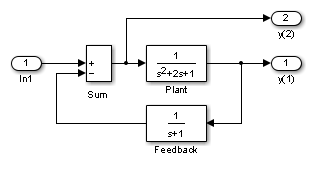## 线性化模型

Simulink® 产品提供 ` linmod``linmod2``dlinmod` 函数来从 Simulink 模型中提取线性状态空间模型。然而，这些函数仅提供基本的线性化功能。要获得完整的线性化功能，请使用 Simulink Control Design™ 软件。有关详细信息，请参阅Choose Linearization Tools (Simulink Control Design)

`linmod``linmod2``dlinmod` 函数采用状态空间矩阵 ABCD 的形式提取线性模型。状态空间矩阵将线性输入-输出关系描述为

`$\begin{array}{l}\stackrel{˙}{x}=Ax+Bu\\ y=Cx+Du,\end{array}$````[A,B,C,D] = linmod('lmod') A = -2 -1 -1 1 0 0 0 1 -1 B = 1 0 0 C = 0 1 0 0 0 -1 D = 0 1 ```

• 转换为 LTI 对象

```sys = ss(A,B,C,D); ```
• 波特相位和幅值频率图

```bode(A,B,C,D) or bode(sys) ```
• 线性化的时间响应

```step(A,B,C,D) or step(sys) impulse(A,B,C,D) or impulse(sys) lsim(A,B,C,D,u,t) or lsim(sys,u,t) ```

```[A,B,C,D] = linmod('sys', x, u) ```